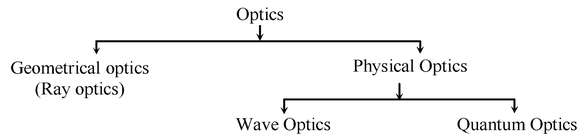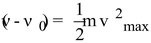# Light

## Light of Class 10

Light is a form of electromagnetic energy that causes the sensation of vision.

### NATURE OF LIGHT

The study of light is called optics and is classified in the following ways:### OPTICS:

It is the branch of physics which deals with the study of light. It is mainly divided into three parts:

• Geometrical optics or ray optics: It deals with the reflection or refraction of light.
• Wave or physical optics: It is concerned with nature of light and deals with interference, diffraction and polarization.
• Quantum optics: It deals with the interaction of light with the atomic entities of matter such as Photo Electric effect, atomic excitation etc.

### PARTICLE THEORY OF LIGHT:

According to Newton, light travels in space with a great speed as a stream of very small particles called corpuscles. This theory failed to explain the interference of light as well as the diffraction of light. So a wave theory of light was accepted.

### WAVE THEORY OF LIGHT:

According to the wave theory, light consists of electromagnetic waves. The light waves travel with a very high speed of 3 × 108 m/s in vacuum.

The wavelength of visible light waves lies between 4 × 10–7 m to 8 × 10–7 m.

The phenomenon of diffraction (bending of light around the corners of tiny objects) interference and polarization of light can only be explained if the light is considered to be of wave nature.

### MODERN QUANTUM THEORY OF LIGHT:

When light falls on the surface of metals like Caesium, Potassium etc., Electrons are thrown out. These electrons are called ‘photo-electrons’ and the phenomenon is called ‘photo-electric effect'.

This was explained by Einstein. According to Planck, light consisted of packets or quanta of energy called photons. Plank also studied this problem much earlier and constructed a mathematical model, according to which light consists of packets or quantas of energy. Einestein explained this later in 1905. He named a quantum of energy as ‘Photon’. The rest mass of photon is zero. Each quanta carries energy E= hv.

v → Planck’s constant = 6.6 × 10-34 J-s.

v → frequency of light

Einstein’s photo-electric equation

h.

hνo = amount of energy spent in ejecting and electron out of metal surface.

Vmax= maximum velocity of the ejected electron.

Some phenomenon’s like interference of light, diffraction of light are explained with the help of wave theory but wave theory failed to explain the photo electric effect. It was explained with the help of quantum theory. So, light has dual nature.

1. Wave nature
2. Particle nature

Related Topics